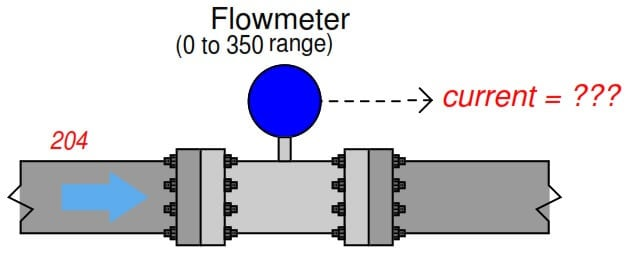Home » Calculate Flow Transmitter Output Current

Calculate Flow Transmitter Output Current

A  flow transmitter  is  ranged  0  to  350  GPM,   4-20  mA  output,   direct-responding. Calculate  the current signal value at a flow rate  of 204 GPM.We are solving this problem using standard 4-20mA conversion Formula. Click here for formula

One  way  we could  solve for the amount  of signal  current  is to convert  the flow value  of 204 .

GPM  into a ratio of the flowmeter’s full-flow value,  then apply  the same  formula  we used  in the previous example relating percentage to milliamps.  Converting the flow rate into a “per unit” ratio is a matter of simple division, since the flow measurement range is zero-based:Therefore,  the transmitter should output a PV signal of 13.3 mA at a flow rate of 204 GPM.

Flow Transmitter Calculations

An alternative approach is to set  up  a linear  equation specifically for this flowmeter given its measurement range (0 to 350 GPM)  and output signal range (4 to 20 mA). We will begin this process by sketching  a simple graph  relating flow rate to current:Therefore,  the transmitter should output a PV signal of 13.3 mA at a flow rate of 204 GPM.

We can also solve the problem using direct formula. Click here for formula

Articles You May Like :

Flow Control Loop Controller

Variable Area Flow Meters

Linear to Square Root Extraction

PV to Percentage Conversion

Closed Tank Level Calculations

Example of 3-15 psi to 4-20mA ConversionOctober 2, 2015 at 7:24 pm

Also Another Simple way to calculate:
Signal = 4 – 20 mA..
So Signal varies between 16 mA
So Each gallon tends to have (16/350)= 0.046 mA
So 204 Gallon will make (0.0457 * 204) = 9.33 mA
Hence Output signal will be 4 mA + 9.33 mA = 13.33 mANovember 22, 2016 at 7:33 am

how calibrate a ff type flow transmitter ABB 2600T range is 53.39mbar and out showing 5000m3/H how calculate plz gv reply on transmitter showing 53.39 m baar and on data sheet showing calibration range 5000 m3/hm confuse

This website uses cookies to improve your experience. We'll assume you're ok with this, but you can opt-out if you wish. Accept Read More

WordPress Image Lightbox

Send this to a friend